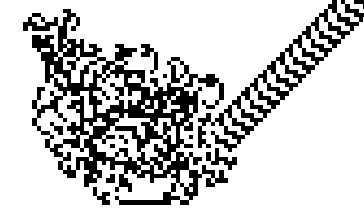# 兰顿蚂蚁

兰顿蚂蚁是元胞自动机的例子，其思路可以被用于元胞自动机，其原理大同小异。下面开始介绍：
Wikipedia

## 介绍

“（兰顿蚂蚁）由克里斯托夫·兰顿在1986年提出，它由黑白格子和一只“蚂蚁”构成，是一个二维图灵机。兰顿蚂蚁拥有非常简单的逻辑和复杂的表现。在2000年兰顿蚂蚁的图灵完备性被证明。兰顿蚂蚁的想法后来被推广，比如使用多种颜色。”

下面展示的是最简单的兰顿蚂蚁。

其中用MATLAB的实现也相对容易。

switch列举出所有的可能性与方案

## 代码

``````function Langton_ant(matrix)
figure;
input('Continue?');
siz = size(matrix);
ori = [floor(siz(1)/2), floor(siz(2)/2)];
direction = 0;
% 方向记为0,1,2,3

Ii = imshow(matrix);

while true
switch direction
case 0
ori(1) = ori(1) + 1;
case 1
ori(2) = ori(2) + 1;
case 2
ori(1) = ori(1) - 1;
case 3
ori(2) = ori(2) -1;
otherwise
end
% direction决定横纵坐标的增减

for i=1:2
if 1>ori(i)
ori(i) = siz(i);
elseif ori(i)>siz(i)
ori(i) = 1;
end
end

if matrix(ori(1),ori(2)) == 0
direction = mod(direction+1, 4);
matrix(ori(1),ori(2)) = 1;
else
direction = mod(direction-1, 4);
matrix(ori(1),ori(2)) = 0;
end
% 前行方向确定

set(Ii, 'CData', matrix);
pause(0.0001);
end
end
``````

以下是模拟结果：不仅兰顿蚂蚁如此，我看过的模拟交通的代码，程序逻辑同样相对简单，不同的是每一辆车多出许多属性，如速度等，只要细心列举出所有的条件，那么MATLAB的实现也是简单的。